# Kerr metric

(diff) ← Older revision | Latest revision (diff) | Newer revision → (diff)

The solution of the Einstein equation describing the external gravity field of a rotating source with massand angular momentum. It is of typeaccording to the classification of A.Z. Petrov. The simplest description is as the Kerr–Schild metric: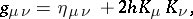where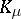is the null vector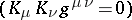, tangent to the special principal null congruence with rotation (of non-gradient type), and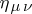is the metric tensor of Minkowski space. The characteristic parameter of the Kerr metric is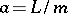. In the general case in the presence of a charge(a Kerr–Newman metric) the scalar functionhas the formwhere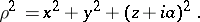The field is singular on the annular thread of radius(when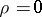). For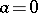the singularity contracts to a point; when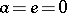the Kerr metric becomes the Schwarzschild metric.

The Kerr metric was obtained by R.P. Kerr .

How to Cite This Entry:
Kerr metric. Encyclopedia of Mathematics. URL: http://encyclopediaofmath.org/index.php?title=Kerr_metric&oldid=17370
This article was adapted from an original article by A.Ya. Burinskii (originator), which appeared in Encyclopedia of Mathematics - ISBN 1402006098. See original article